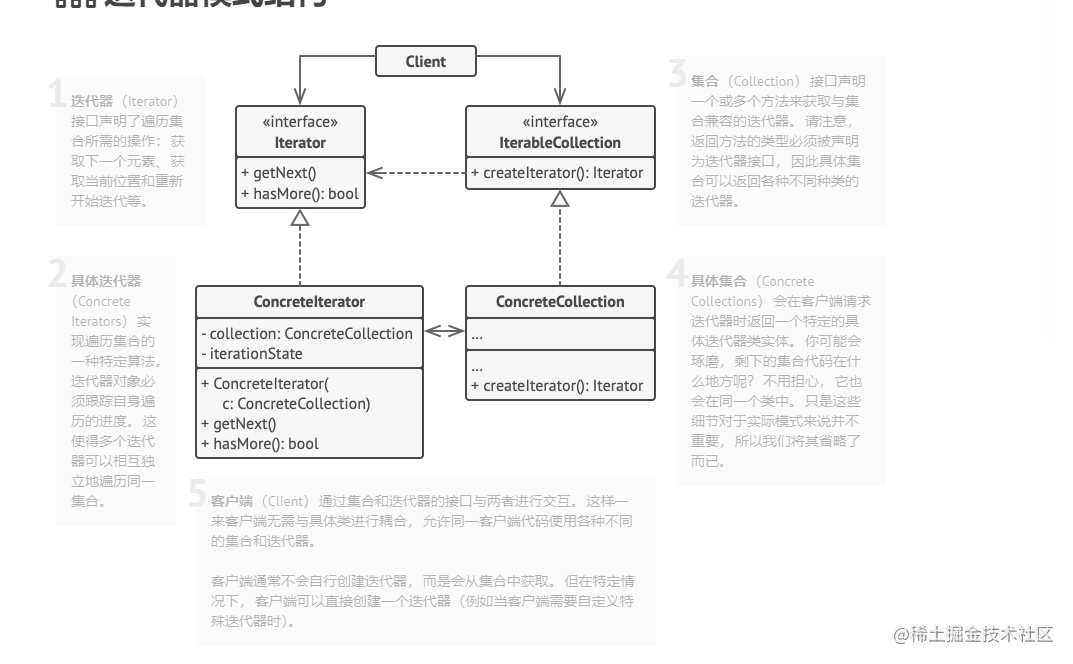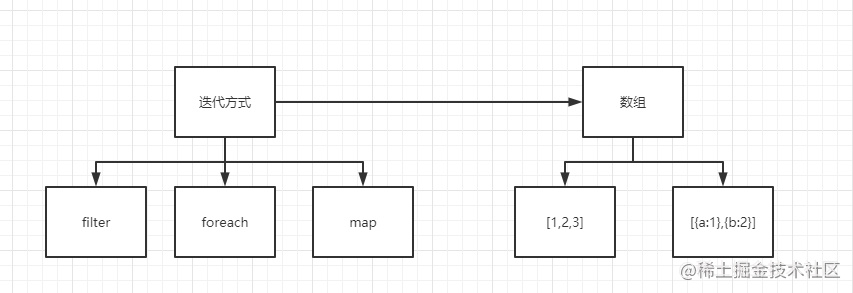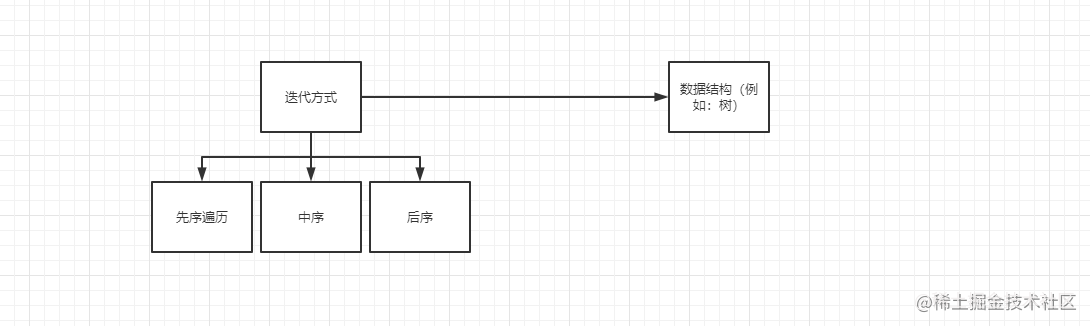# 迭代器模式

1. ##### 迭代

迭代或者说遍历是我们日常开发经常会遇到的一个场景，当遇到数组或者对象等数据结构时我们总会用上

2. ##### 迭代器模式概念

迭代器模式主要的思想就是在在不暴露集合底层表现形式 （列表、 栈和树等） 的情况下遍历集合中所有的元素。1. ##### 特点
迭代方式和数据源的分离解耦1. ##### 案例
• forEach就是一种按顺序遍历的迭代方式

``````const array = [1,2,3];
array.forEach(item => {console.log(item)}) // 1,2,3
复制代码``````
• 具体的迭代的实现

``````// 按顺序
Array.prototype.forEach = function(fn) {
for(var i=0; i < this.length; i++){
fn(this[i])
}
}
// 反着遍历
Array.prototype.forEach_reverse = function(fn) {
for(var i= this.length - 1; i >= 0; i--){
fn(this[i])
}
}
array.forEach_reverse(item => {console.log(item)}) // 3,2,1

// map,filter,some,every.....
复制代码``````
• ES6 的 Iterator 迭代器 （for of的原理）

ES6 中迭代器 Iterator 作为一个接口，作用就是为各种不同数据结构提供统一的访问机制。任何数据结构只要部署了 Iterator 接口，就可以完成遍历操作。

ES6 默认的 Iterator 接口部署在数据结构的 Symbol.iterator 属性上，该属性本身是一个函数，代表当前数据结构默认的遍历器生成函数。执行该函数 `[Symbol.iterator]()`，会返回一个遍历器对象。只要数据结构拥有 Symbol.iterator 属性，那么它就是 “可遍历的” 。

``````function getIterator(list) {
var i = 0;
return {
next: function() {
var done = (i >= list.length);
var value = !done ? list[i++] : undefined;
return { done: done, value: value };
}
};
}

let arr = ['a', 'b', 'c'];
let iterator = arr[Symbol.iterator]();

iterator.next();  // { value: 'a', done: false }
iterator.next();  // { value: 'b', done: false }
iterator.next();  // { value: 'c', done: false }
iterator.next();  // { value: undefined, done: true }
复制代码``````
• Map 迭代器

``````const citys = new Map([['北京', 1], ['上海', 2], ['杭州', 3]])
const run = citys.entries()

run.next() // {value: ['北京', 1], done: false}
run.next() // {value: ['上海', 2], done: false}
run.next() // {value: ['杭州', 3], done: false}
run.next() // {value: undefined, done: true}
复制代码``````1. ##### 总结

迭代器模式可以把迭代的过程从业务逻辑中分离出来，在使用迭代器模式之后，即使不关心对象的内部构造，也可以按特定的方法访问其中的每个元素。